# 多个变量的线性回归问题【机器学习】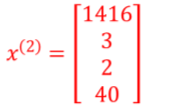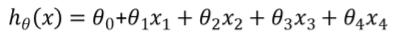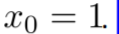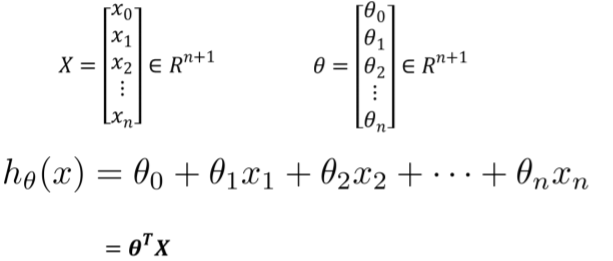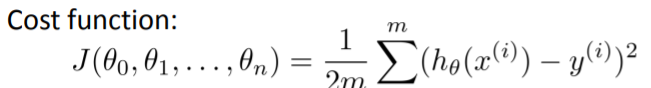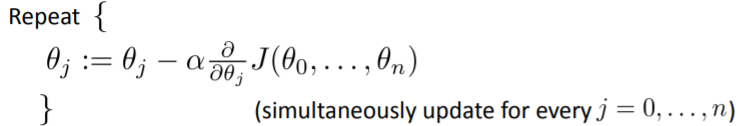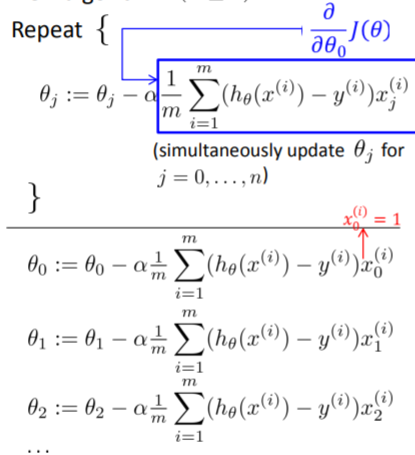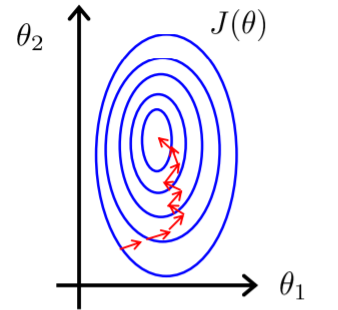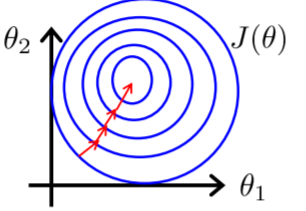$x_i = \frac{x_i - u_i}{s_i}$

$x = \frac{x - min}{max = min}$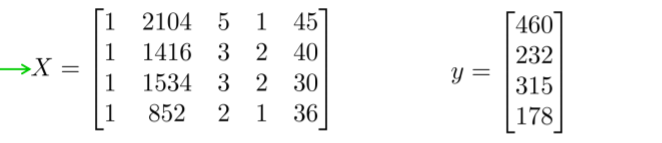$X\theta = y$

$X^TX\theta = yX^T => \theta = (X^TX)X^Ty$